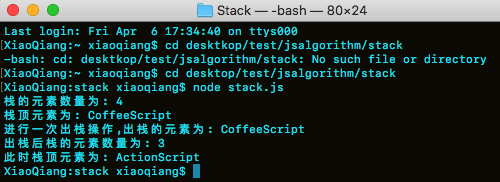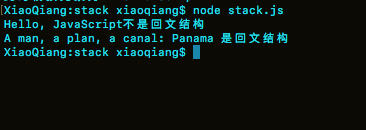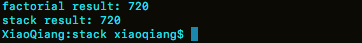# JavaScript数据结构与算法——栈详解

### 2、JS中栈的实现

#### 实现栈构造函数

function Stack() {
this.stack = []
this.top = 0
this.pop = pop
this.push = push
this.peek = peek
this.length = length // 用于返回栈元素数量
this.clear = clear // 用于清空栈
}

#### push()

function push(param) {
this.stack[this.top++] = param
}

#### pop()

function pop() {
return this.stack[--this.top]
}

#### peek()

function peek() {
return this.stack[this.top - 1]
}

#### length()

function length() {
return this.top
}

function clear() {
this.top = 0
}

### 测试实现的栈

#### 测试用例

var stack = new Stack()
stack.push('JavaScript')
stack.push('TypeScript')
stack.push('ActionScript')
stack.push('CoffeeScript')
console.log('栈的元素数量为: ' + stack.length())
console.log('栈顶元素为: ' + stack.peek())
console.log('进行一次出栈操作,出栈的元素为: ' + stack.pop())
console.log('出栈后栈的元素数量为: ' + stack.length())
console.log('此时栈顶元素为: ' + stack.peek())


#### 测试结果### 3、栈应用举例

#### 3.1、回文问题

function isPalindrome(str) {
// 新建一个栈
var stack =  new Stack()
// 使用正则表达式取出句子中的字母
str = str.replace(/\W/g, '').toLowerCase();
// 将所有字母入栈
for (var i = 0; i < str.length; i++) {
stack.push(str[i])
}
// 出栈操作, 拼接每次出栈的字母
var reverse = ''
while (stack.length() > 0) {
reverse += stack.pop()
}
// 如果入栈顺序和出栈顺序一致, 则为回文结构
if (str === reverse) {
return true
} else {
return false
}
}

// test
var str = 'Hello, JavaScript'
if (isPalindrome(str)) {
console.log(str + ' 是回文结构')
} else {
console.log(str + '不是回文结构')
}

var str1 = 'A man, a plan, a canal: Panama'
if (isPalindrome(str1)) {
console.log(str1 + ' 是回文结构')
} else {
console.log(str1 + '不是回文结构')
}

node stack.js 得到以下结果，证明革命成功：#### 3.2、模拟递归

// 递归问题
function factorial(num) {
if (num === 0) {
return 1
} else {
return num * factorial(num - 1)
}
}
console.log(factorial(6))

5的阶乘为5x4x3x2x1，那么我们把54321都推进栈里，然后在出栈时计算阶乘应该就可以了吧？试试：

function stackFactorial(num) {
var s = new Stack()
while (num > 1) {
s.push(num--)
}
var result = 1
while (s.length() > 0) {
result *= s.pop()
}
return result
}
console.log('stack result: ' + stackFactorial(6))

node stack.js 得到以下结果：